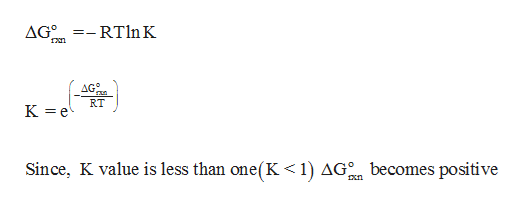# A student determines the value of the equilibrium constant to be 62×10-18for the following reaction.Fe3O4(s) + 4H2(g)3Fe(s) + 4H2O(g)Based on this value of Keq:G° for this reaction is expected to be (greater, less)  _____ than zero. Calculate the free energy change for the reaction of 1.89 moles of Fe3O4(s) at standard conditions at 298K.     G°rxn =  ______ kJ

Question
1. A student determines the value of the equilibrium constant to be 62×10-18for the following reaction.

Fe3O4(s) + 4H2(g)3Fe(s) + 4H2O(g)

Based on this value of Keq:

G° for this reaction is expected to be (greater, less)  _____ than zero.

Calculate the free energy change for the reaction of 1.89 moles of Fe3O4(s) at standard conditions at 298K.
G°rxn =  ______ kJ
check_circleExpert Solution
Step 1

From the relationship between equilibrium constant (K) and ΔG0, it is clear that when the value of K becomes less than one, then G° for the reaction is expected to be less than zero.help_outlineImage Transcriptionclose=- RT ln K AG0 AG° RT K e Since, K value is less than one(K < 1) AG becomes positive fullscreen
Step 2

The value of ∆G per mole for the reaction can be determined using the given formula as follows,

Step 3

The value of ∆G per mole for the reaction is 92.46 kJ/mol. There are 1.89 moles of Fe3O4 presen...

### Want to see the full answer?

See Solution

#### Want to see this answer and more?

Solutions are written by subject experts who are available 24/7. Questions are typically answered within 1 hour*

See Solution
*Response times may vary by subject and question
Tagged in

### Chemistry